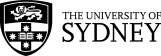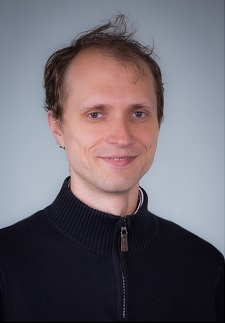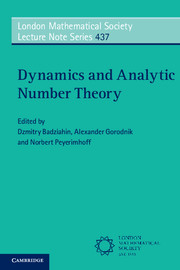School of Mathematics and Statistics

## Contact details

Office C634
School of Mathematics and Statistics F07
University of Sydney NSW 2006
Australia

Phone: +61 2 9351 3279## Research

My research interests lie mostly in Analytical Number theory, in particular Diophantine approximation. More specific areas of interest:

• Various types of badly approximable sets
• Generalised Cantor-type sets
• Littlewood conjecture and its analogue.
• Approximational properties of Mahler functions and numbers
I'm also interested in some aspects of combinatorics on words, computational number theory, dynamic systems and ergodicity.

## Publications

### Edited BookD. Badziahin, A. Gorodnik and N. Peyerimhoff, Dynamics and Analytic Number theory, London Mathematical Society Lecture Note Series 437, Cambridge University Press, 2016. Written by leading experts, this book explores several directions of current research at the interface between dynamics and analytic number theory. Topics include Diophantine approximation, exponential sums, Ramsey theory, ergodic theory and homogeneous dynamics. The origins of this material lie in the 'Dynamics and Analytic Number Theory' Easter School held at Durham University in 2014. Key concepts, cutting-edge results, and modern techniques that play an essential role in contemporary research are presented in a manner accessible to young researchers, including PhD students. This book will also be useful for established mathematicians. The areas discussed include ubiquitous systems and Cantor-type sets in Diophantine approximation, flows on nilmanifolds and their connections with exponential sums, multiple recurrence and Ramsey theory, counting and equidistribution problems in homogeneous dynamics, and applications of thin groups in number theory. Both dynamical and 'classical' approaches towards number theoretical problems are also provided.

### Preprints

With J. Schleischitz, An improved bound in Wirsing's problem.

With Y. Bugeaud, Multiplicative p-adic approximation.

With S. Harrap, E. Nesharim, D. Simmons, Schmidt games and Cantor winning sets.

### In Refereed Journals

On spectrum of irrationality exponents of Mahler numbers. J. de Theorie des Nombres de Bordeaux, V 31(2), pp. 431 -- 453, 2019.

Continued fractions of certain Mahler functions, Acta Arithmetica, V. 188(1), pp. 53 -- 81, 2019.

With E. Zorin, On generalized Thue-Morse functions and their values, J. of AustMS., 2019.

With E. Zorin, On irrationality measure of Thue-Morse constant, Math. Proc. Cambridge Philos. Soc., 2018.

With S. Harrap, Cantor-winning sets and their applications, Advances in Math., V. 318, pp. 627 -- 677, 2017.

With S. Harrap, M. Hussain, An Inhomogeneous Jarnik type theorem for planar curves, Math. Proc. Cambridge. Philos. Soc., V 163(1), pp. 47 -- 70, 2017.

Finding special factors of values of polynomials at integer points, Int. J. of Numb. Theor., V. 13(1), 2017.

Continuant Diophantine equations, Moscow J. of Comb. and Numb. Theor., V 6(2--3), pp. 38 -- 55, 2016.

Computation of the Infimum in the Littlewood Conjecture, Experimental Math., V. 25(1), pp. 100 -- 105, 2016.

With J. Shallit, An Unusual Continued Fraction, Proc. Amer. Math. Soc., V. 144(5), pp. 1887 -- 1896, 2015.

With Y. Bugeaud, M. Einsiedler, D. Kleinbock, On the complexity of a putative counterexample to the p-adic Littlewood conjecture, Compositio Math., V. 151(9), pp. 1647 -- 1662, 2015.

With E. Zorin, Thue-Morse constant is not badly approximable, Int. Math. Res. Notes., V. 2015(19), pp. 9618 -- 9637, 2015.

With S. Velani, Badly approximable points on planar curves and a problem of Davenport , Math. Annalen, V. 359(3--4), pp. 969 -- 1023, 2014.

On multiplicatively badly approximable numbers, Mathematika, V. 59(1), pp. 31 -- 55, 2013.

With V. Beresnevich and S. Velani, Inhomogeneous theory of dual Diophantine approximation on manifolds, Advances in Math., V. 232, pp. 1 -- 35, 2013.

With S. Velani. Multiplicatively badly approximable numbers and generalised Cantor sets, Advances in Math., V. 228(5), pp. 2766 -- 2796, 2011.

With A. Pollington and S. Velani, On a problem in simultaneous Diophantine approximation: Schmidt's conjecture, Annals of Math., V. 174, pp. 1837 -- 1883, 2011.

With J. Levesley and S. Velani, The mixed Schmidt conjecture in the theory of Diophantine approximation, Mathematika, V. 57(2), pp. 239 -- 245, 2011.

With A. Haynes, A note on Farey fractions with denominators in arithmetic progressions, Acta Arithmetica, V. 147(3), pp. 205 -- 215, 2011.

Inhomogeneous Diophantine approximation on curves and Hausdorff dimension, Advances in Math., V. 223(1), pp. 329 -- 351, 2009.

With I. Morozova, On the exact exponent of convergence of series with small denominators. Vestsi Nats. Akad. Navuk Belarusi Ser. Fiz.-Mat. Navuk 2007, N. 2, pp 37 -- 40. (in Russian)

With J. Levesley, Convergence results for simultaneous and multiplicative Diophantine approximation on planar curves, Glasgow Math. J., V. 49, pp. 367 -- 375, 2007.

With J. Levesley, Computation of fractional measures in the case of convergence for Diophantine approximations on planar curves. Dokl. Nats. Akad. Nauk Belarusi V. 50, N. 6, pp. 24--27, 2006. (in Russian)

Inhomogeneous Diophantine approximation and lower bounds of Hausdorff dimension, Vesti Nats. Acad. Navuk Belarusi. Ser. Fiz.-Mat. Navuk 2005, N. 3, pp 32 -- 36. (in Russian)

With I. Morozova, On small denominators of product of linear forms, proceeding of the international mathematical conference in honor of Skorobogatko, Lvov 2004. (in Russian)

## Some other math. related interests

I hold the position of AMOC state director for New South Wales.
I organise the Tournament of the Towns in Sydney. Useful information about the tournament.

Here are the computational projects in which I participated:

• Finding Mersenne primes: GIMPS
• Factoring Fermat numbers: www.fermatsearch.org
• Finding Aliquot cycles (I found 17 new cycles of length more than two and about a thousand new cycles of length two): here is the link to all known cycles of length greater than two.

## Hobby

My most favorite hobbies are:
• Hiking and travelling. Here are some photos: one, two, three.
• Programming. Here is a webpage with Kvetka chess application.